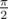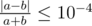# Cut the pie

Arkady reached the n-th level in Township game, so Masha decided to bake a pie for him! Of course, the pie has a shape of convex n-gon, i.e. a polygon with n vertices.

Arkady decided to cut the pie in two equal in area parts by cutting it by a straight line, so that he can eat one of them and give the other to Masha. There is a difficulty because Arkady has already put a knife at some point of the pie, so he now has to cut the pie by a straight line passing trough this point.

Help Arkady: find a line that passes through the point Arkady has put a knife into and cuts the pie into two parts of equal area, or determine that it’s impossible. Your program has to quickly answer many queries with the same pie, but different points in which Arkady puts a knife.

Input

The first line contains two integers n and q (3 ≤ n ≤ 104, 1 ≤ q ≤ 105) — the number of vertices in the pie and the number of queries.

n line follow describing the polygon vertices in clockwise order. The i-th of these line contains two integers x i and y i ( - 106 ≤ x i, y i ≤ 106) — the coordinates of the i-th vertex. It is guaranteed that the polygon is strictly convex, in particular, no three vertices line on the same line.

An empty line follows.

q lines follow describing the query points. The i-th of these lines contain two integers x i and y i ( - 106 ≤ x i, y i ≤ 106) — the coordinates of the point in which Arkady puts the knife in the i-th query. In is guaranteed that in each query the given point is strictly inside the polygon, in particular, is not on its edges.

Output

For each query print single integer — the polar angle of the line that is the answer for the corresponding query, in radians. The angle should be in the segment [0;π], the angles are measured from the direction of OX axis in counter-clockwise order. For example, the polar angle of the OY axis is. If there is no answer in that query, print -1.

If there are several answers, print any of them. Your answer is considered correct if the difference between the areas of the parts divided by the total area of the polygon doesn’t exceed 10 - 4 by absolute value. In other words, if a and b are the areas of the parts after the cut, then your answer is correct if and only of.

Examples

input

3 10 00 33 01 1

output

2.67794504460098710000

input

5 36 56 35 00 00 55 43 35 2

output

0.602287346126900490001.279339532264735800002.85805511179015910000

Solution:

#include <bits/stdc++.h>

using namespace std;

const double pi = acos(-1.0);

const int N = 1234567;

int x[N], y[N];
int n;
double areas[N];

void line(int i, int j, double &a, double &b, double &c) {
i %= n;
j %= n;
a = y[j] - y[i];
b = x[i] - x[j];
c = -a * x[i] - b * y[i];
}

void intersect(double a, double b, double c, double aa, double bb, double cc, double &dx, double &dy) {
double d = a * bb - b * aa;
dx = (b * cc - c * bb) / d;
dy = (c * aa - a * cc) / d;
}

int main() {
int q;
scanf("%d %d", &n, &q);
for (int i = 0; i < n; i++) {
scanf("%d %d", x + i, y + i);
}
reverse(x, x + n);
reverse(y, y + n);
areas = 0;
for (int i = 0; i < 4 * n; i++) {
areas[i + 1] = areas[i] + (x[i % n] - x[(i + 1) % n]) * 1.0 * (y[i % n] + y[(i + 1) % n]);
}
double total_area = 0.0;
for (int i = 0; i < n; i++) {
total_area += (x[i] - x[(i + 1) % n]) * 1.0 * (y[i] + y[(i + 1) % n]);
}
for (int i = 0; i < q; i++) {
int qx, qy;
scanf("%d %d", &qx, &qy);
double low = 0, high = pi;
double zero = -1.0;
bool found = false;
double ans = -1.0;
for (int it = 0; it < 60; it++) {
double mid = 0.5 * (low + high);
if (mid == low || mid == high) {
break;
}
if (it == 0) {
mid = low;
}
double dx = cos(mid);
double dy = sin(mid);
double a = dy;
double b = -dx;
double c = -a * qx - b * qy;
double u0 = a * x + b * y + c;
double u1 = a * x + b * y + c;
bool flag = false;
if (u0 > u1) {
flag = true;
u0 = -u0;
u1 = -u1;
a = -a;
b = -b;
c = -c;
}
int ll = 0, rr = n - 1;
while (ll < rr) {
int i = (ll + rr + 1) >> 1;
int j = (i + 1) % n;
double ui = a * x[i] + b * y[i] + c;
double uj = a * x[j] + b * y[j] + c;
if (ui <= uj && ui >= 0.5 * (u0 + u1)) {
ll = i;
} else {
rr = i - 1;
}
}
int id_max = (ll + 1) % n;
ll = id_max + 1, rr = id_max + n - 1;
while (ll < rr) {
int i = (ll + rr) >> 1;
int j = i + 1;
double ui = a * x[i % n] + b * y[i % n] + c;
double uj = a * x[j % n] + b * y[j % n] + c;
if (ui > uj) {
ll = i + 1;
} else {
rr = i;
}
}
int id_min = ll;
int last, first;
{
ll = id_max, rr = id_min;
while (ll < rr) {
int i = (ll + rr + 1) >> 1;
double ui = a * x[i % n] + b * y[i % n] + c;
if (ui > 0) {
ll = i;
} else {
rr = i - 1;
}
}
last = ll + n;
}
{
ll = id_min, rr = id_max + n;
while (ll < rr) {
int i = (ll + rr) >> 1;
double ui = a * x[i % n] + b * y[i % n] + c;
if (ui < 0) {
ll = i + 1;
} else {
rr = i;
}
}
first = ll;
}
double xf, yf, xl, yl;
double aa, bb, cc;
line(first - 1, first, aa, bb, cc);
intersect(a, b, c, aa, bb, cc, xf, yf);
line(last, last + 1, aa, bb, cc);
intersect(a, b, c, aa, bb, cc, xl, yl);
double res = 0.0;
res += (xf - x[first % n]) * (yf + y[first % n]);
res += areas[last] - areas[first];
res += (x[last % n] - xl) * (y[last % n] + yl);
res += (xl - xf) * (yl + yf);
if (flag) {
res = total_area - res;
}
if (it == 0) {
zero = res;
continue;
}
{
// checking
double res1 = 0.5 * res;
double res2 = 0.5 * (total_area - res);
double diff = fabs(res1 - res2);
double sum = 0.5 * total_area;
if (diff / sum < 1e-6) {
found = true;
ans = mid;
break;
}
}
if ((res > 0.5 * total_area) == (zero > 0.5 * total_area)) {
low = mid;
} else {
high = mid;
}
}
printf("%.17f\n", found ? ans : (0.5 * (low + high)));
}
return 0;
}